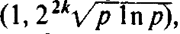# Primitive Root

Also found in: Wikipedia.

## primitive root

[′prim·əd·iv ′rüt]
(mathematics)
An n th root of unity that is not an m th root of unity for any m less than n.
McGraw-Hill Dictionary of Scientific & Technical Terms, 6E, Copyright © 2003 by The McGraw-Hill Companies, Inc.
The following article is from The Great Soviet Encyclopedia (1979). It might be outdated or ideologically biased.

## Primitive Root

A primitive root modulo m is a number g such that the smallest positive number k for which the difference gk — 1 is divisible by m—that is, for which gk is congruent to 1 modulo m—coincides with ɸ(m), where ɸ(m) is the number of positive integers less than m and relatively prime to m. For example, if m = 7, the number 3 is a primitive root modulo 7. In fact, ɸ(7) = 6, since the numbers 31 – 1 = 2, 32 – 1 = 8, 33 - 1 = 26, 34 - 1 = 80, and 35 - 1 = 242 are not divisible by 7—only 36 — 1 = 728 is divisible by 7. Primitive roots exist only for m = 2, m = 4, m = pa, and m = 2pa, where ρ is an odd prime and α is a positive integer. The number of primitive roots in these cases is equal to ɸ[ɸ(m)] (numbers whose difference is divisible by m are not considered distinct). In 1926, 1. M. Vinogradov showed that a primitive root modulo p, where ρ is an odd prime, can be found in the interval, where k is the number of distinct prime divisors of ρ — 1.

### REFERENCES

Vinogradov, I. M. Osnovy teorii chisel, 8th ed. Moscow, 1972.
Vinogradov, I. M. Izbr. trudy. Moscow, 1952. Pages 54–57.
Mentioned in ?
References in periodicals archive ?
Primitive Roots. If "p" is a prime and "a" is any element in the residual set of [Z.sub.p] = {1, 2, 3, ..., p - 1} and if a mod p, [a.sup.2] mod p, [a.sup.3] mod p, ..., [a.sup.p-1] mod p are distinct and consist of integers within the range [1 : p - 1], then "a" is called as the primitive root or generator of "p" [22, 23].
According to this fact, we propose a minimum parity check matrix [H.sub.min] corresponding to primitive root of the codes as follows:
Smarandache function, divisibility, primitive root.
To get a period of maximal length m - 1, m must be a prime; a is a primitive root of m; and [x.sub.0] [Epsilon][1, m - 1] (cf.
If [per.sub.n](a) = [phi](n), we say that a is a primitive root (mod n).
The generator advocated by Park and Miller  as a "minimal standard" random number generator is the congruential generator [X.sub.n] = 16807[X.sub.n] - 1 mod p for the prime modulus p = [2.sup.31] - 1, or more generally, [X.sub.n] = [ax.sub.n] - 1 mod p for any primitive root a of p.
Let c be a primitive root for r, let [chi] be a character of order n defined by [chi](c) = [omega] where [omega] = [e.sup.2[pi]i/n] and let [MATHEMATICAL EXPRESSION NOT REPRODUCIBLE IN ASCII] be the Gauss sum of [chi] where [F.sub.r] is a finite field of order r.
Abstract Let [prim.sub.[??]](x) denote the number of square-free primitive roots not exceeding x modulo p and let [g.sub.[??]](p) denote the smallest square-free primitive root modulo p.
(6) a.sup.m-1 mod m = 1 (7) and a standard number theoretic definition: If m is prime then a is a primitive element modulo m (or primitive root of m) iff a.sup.n mod m [is not =] 1 for n = 1, 2, ..., m - 2 [18, p.
By Horie [4, Theorem 2], l [??] [h.sup.-.sub.n]/[h.sup.-.sub.n-1] for all n [greater than or equal to] 1 if l is a primitive root modulo [p.sup.2] and l is larger than an explicit but complicated constant depending on p.
Among specific topics are the prime numbers, congruences, primitive roots, the Hasse-Minkowski theorem, continued fractions, and hyperbolas and Pell's equation.
So F has as eigenvalues the 2520th primitive roots of unity, with multiplicity 1.

Site: Follow: Share:
Open / Close